# Reading Large Numbers in the Millions and Billions

Reading Large Numbers in the Millions and Billions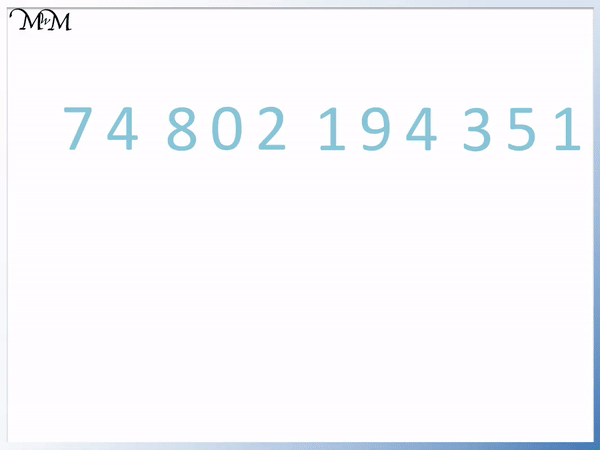• Separate the number into groups of 3 digits.
• Starting from the right and moving left, we have the following group names:
• ‘Hundreds, Tens and Units’, ‘Thousands’, ‘Millions’ and ‘Billions’.
• Read the digits from left to right, starting with the billions group.
• Read each group like the hundreds, tens and units group with the group name afterwards.
• Read the groups from left to right as a list of words.
• Write ‘and’ between the ‘tens and units’ part of each group.
• Notice that we put a hyphen ‘-‘ between every word ending in ‘y’ and the following word.

Group digits of 3 as billions, millions, thousands and HTU.

Read each group as HTU, with the group name following it.• Group the digits into groups of 3 from right to left as HTU, Thousands, Millions and Billions.
• Read these place value groups in words as Hundreds, Tens and Units, from left to right.
• There is 625 in the billions group, so six hundred and twenty-five billion.
• There is 338 in the millions group, so three hundred and thirty-three million.
• There is 45 in the thousands group, so forty-five thousand.
• There is 9 in the HTU group, so we have nine.
• We read the total large number in words as a list from left to right.
• Six hundred and twenty-five billion, three hundred and thirty-three million, forty-five thousand and nine.
• Put a hyphen ‘-‘ between words ending in ‘y’ and the next word.
• Put a comma ‘,’ between each place value group.
• Write ‘and‘ before every ‘tens and units’ part in each group.# How to Write Large Numbers in the Millions and Billions in Words

Digits are any of the numerals: 0, 1, 2, 3, 4, 5, 6, 7, 8 or 9 that are written to make a number.

A number with 3 digits contains hundreds, tens and units.

A number with 4-6 digits is a number in the thousands.

A number with 7-9 digits is a number in the millions.

A number with 10-12 digits is a number in the billions.

In this lesson we are learning how to write large numbers in the millions and billions using words.

To write a large number in words use the following steps:

1. Separate the number into groups of 3 digits.
2. Label these groups from right to left as: HTU, Thousands, Millions and then Billions.
3. Read each group from left to right as though they are hundreds, tens and units but with their group name afterwards.
4. Write a hyphen between any word ending in ‘y’ and the following word.
5. Write a comma between each group in the list and write ‘and’ before the ‘tens and units’ part of each group.

We will use this guide to writing numbers in words with some examples below.

In the first example we will read the number 625 338 045 009.

Numbers are normally written with a space between every three digits, such as in this example. This can help us to separate the number into groups of 3 digits when reading it.

Sometimes commas are used to separate the groups of three and you might see this number written as 625, 338, 045, 009.We put each group of 3 digits into the groups: HTU, Thousands, Millions and Billions from right to left.

We read each group in the same way that we read the HTU group:

• The first digit is the number of hundreds
• Write a hyphen between words ending in ‘y’ and the next word.
• Write ‘and’ between the hundred and the ‘tens and units’ part of the word in each group.

We have 625 in the billions, which is read in words as six hundred and twenty-five billion.

We have 338 in the millions, which is read in words as three hundred and thirty three million.

We have 45 in the thousands, which is read in words as forty-five thousand.

We have 9 in the HTU group, which is read in words as nine.

Putting this list of words together would read as:

Six hundred and twenty-five billion, three hundred and thirty three million, forty-five thousand, nine.

Remember that we need to write ‘and‘ before the list word in the list. We write ‘and‘ before the ‘nine’ in the list above.

This large number written in words is:

Six hundred and twenty-five billion, three hundred and thirty three million, forty-five thousand and nine.We will look at another example of writing a number in the billions in words. Again we will use a place value chart.

We will write the number 74 802 194 351 in words.

We make groups of 3 digits and read from left to right.We have 74 in the billions, which is read in words as seventy-four billion.

We have 802 in the millions, which is read in words as eight hundred and two million.

We have 194 in the thousands, which is read in words as one hundred and ninety-four thousand.

We have 351 in the HTU group, which is read in words as three hundred and fifty-one.

This large number written in words is:

Seventy-four billion, eight hundred and two million, one hundred and ninety-four thousand, three hundred and fifty-one.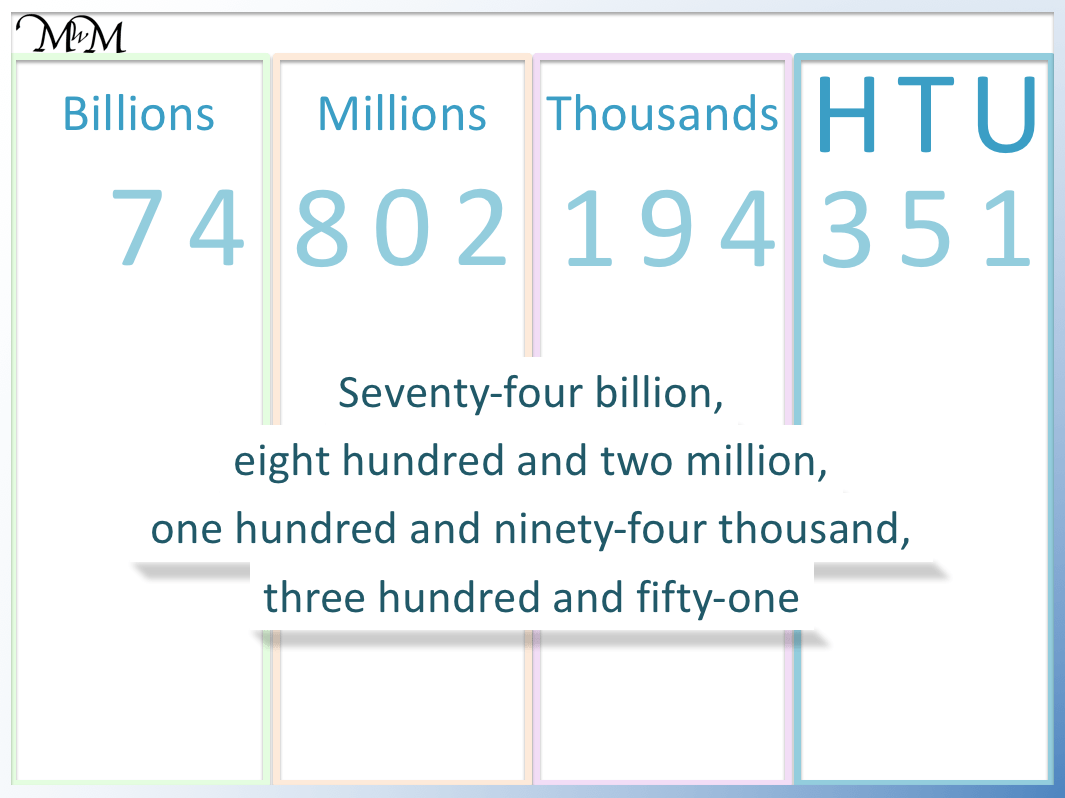In this example we will read the number 148 006 555 327 in words.We have 148 in the billions, which is read in words as one hundred and forty-eight billion.

We have 006 in the millions, which is read in words as six million.

We have 555 in the thousands, which is read in words as five hundred and fifty-five thousand.

We have 327 in the HTU group, which is read in words as three hundred and twenty-seven.

This large number read in words is:

One hundred and forty-eight billion, six million, five hundred and fifty-five thousand, three hundred and twenty-seven.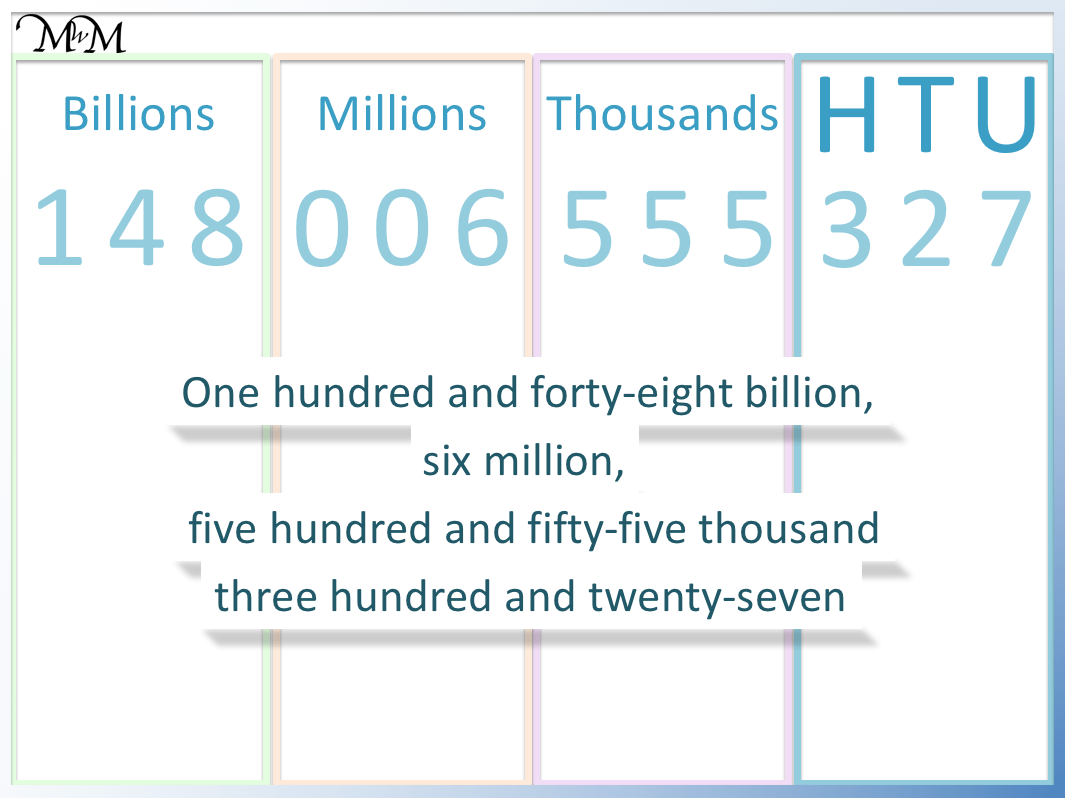Notice that any zero digits are simply ignored.

The next example of writing a number in the billions in words is 591 000 002 895.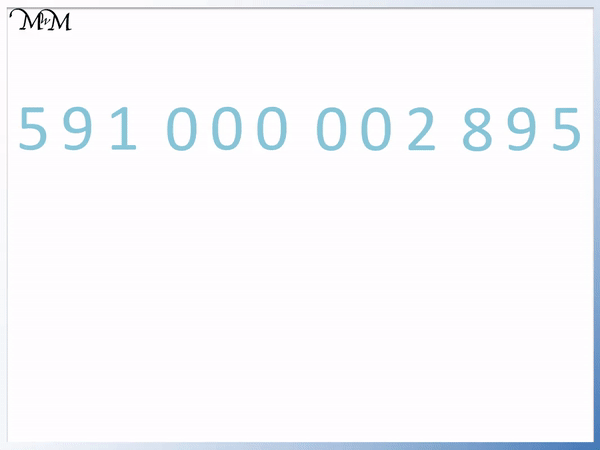We have 591 in the billions, which is read in words as five hundred and ninety-one billion.

We have 000 in the millions, which is not read at all because there are no significant digits. We will skip the millions group when reading it.

We have 002 in the thousands, which is read in words as two thousand.

We have 895 in the HTU group, which is read in words as eight hundred and ninety-five.

This large number read in words is:

Five hundred and ninety-one billion, two thousand, eight hundred and ninety-five.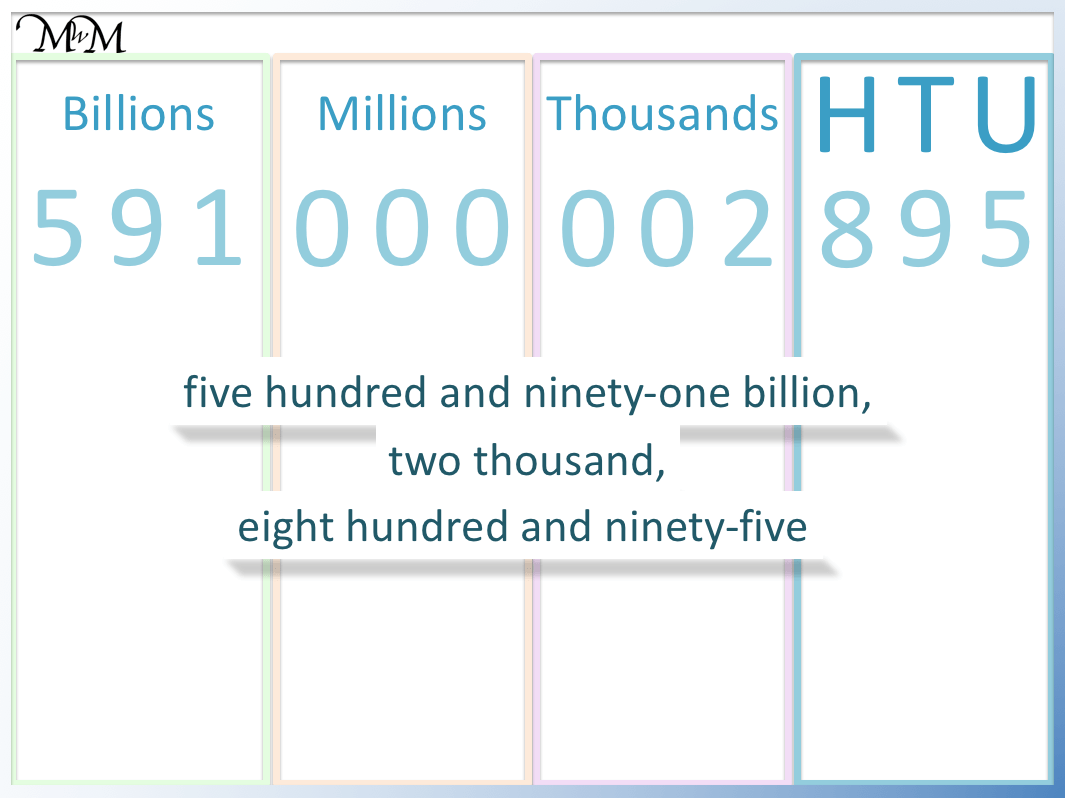Now try our lesson on Place Value of Tenths and Hundredths where we introduce the idea of decimals.error: Content is protected !!